# Maths - Algegraic Topology

 On these pages we discuss some concepts in algegraic topology. Click on the subject on the right to go to the subject you are interested in.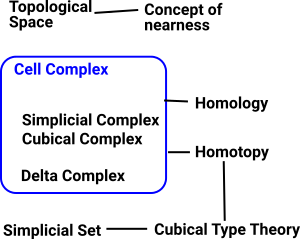## Equality Between Spaces

In two dimensions it is relatively easy to determine if two spaces are topologically equivalent (homeomorphic). We can check if they:

• are connected in the same way.
• have the same number of holes.

However, when we scale up to higher dimensions this does not work and it can become impossible to determine homeomorphism. There are methods which will, at least, allow us to prove more formally when topological objects are not homeomorphic.

These methods use 'invariants': properties of topological objects which do not change when going through a homeomorphism. Here we look at two types of invariants which arise from homotopy and homology.

### Homotopy

It would take up too much space to properly explain the concept here so see this page for more detailsIn homotopy we use equivalence classes between a circle and loops (which don't collapse) on the topological object that we are investigating. We can get further invariants by extending the circle to the surface of higher order n-spheres.

We can then get algebraic structures (mostly groups) by investigating what happens when these loops are composed, the loops are generators of the group. In homotopy the order of these compositions can be significant, that is the groups are not necessarily abelian.

### Homology

It would take up too much space to properly explain the concept here so see this page for more detailsIn homology we dont just use n-spheres but every closed oriented n-dimensional sub-manifold.

It also uses a different definition of equivalence classes where composition of loops commutes. This results in abelian groups.

So we dont need to fix the basepoint.

### Topology on a Set

A topology on a set X is a collection Τ of subsets of X, called open sets satisfying the following properties:

• X and Ø are elements of Τ.
• Τ is closed under finite intersections.
• Τ is closed under arbitrary unions.

The requirements for the existence of of meets and joins correspond to the requirements for the existence of unions and intersections of open sets. Therefore these lattice structures can represent topologies.

Lattice Open Set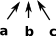invalid - every intersection should be an open set:Venn Diagram Topological Space (or not) Lattice (frame)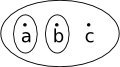This is not a topological space because 'a' and 'b' are subsets but not the union of 'a' and 'b'This is now a topological space because we have added the union of 'a' and 'b'This is not a topological space because 'ab' and 'bc' are subsets but not their intersection.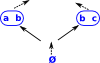This is now a topological space because we have added 'b'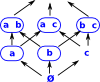Note: Assume Ø is included in the above examples.

## Link between Topology and Logic

 One way to visualise the link between topology and logic is to start with a Venn diagram. We can then map points in the Venn diagram to either true or false depending on whether they are in a given set.This is a nice way to link geometry, logic and topology.

See page here.

See page here.

## Examples

### Non-Geometric Examples

#### Sequences

Here the concept of 'nearness' comes from sequences that begin in the same way.